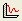Join one of our upcoming courses taking place around the world! Explore now

# Convergence Plot

The Convergence Plot option in the Statistics menu, allows you to view the progress of a Probabilistic Analysis, to determine whether or not the analysis results are converging to constant values, and the approximate number of samples at which convergence occurs. This can help to determine:

• if you have used enough samples for the Probabilistic Analysis, or
• if you can use fewer samples, and still obtain valid results.

To create a Convergence Plot:

1. Select Convergence Plotfrom the toolbar or the Statistics menu.
2. In the Convergence Plot dialog:
• Select the Analysis Method for which you would like to view results.
• Select the data that you would like to plot (see below for details)
3. Select the Plot button to generate the plot.
4. Once a plot is generated, there are several options available, if you right-click the mouse on the plot. See below for details.

## Convergence Plot

The following analysis results can be plotted on a Convergence Plot:

• Factor of Safety (mean)
• Factor of Safety (standard deviation)
• Factor of Safety (minimum)
• Probability of Failure (mean)
• Probability of Failure (standard deviation)

The mean and standard deviation plots (Factor of Safety, or Probability of Failure), are obtained by recalculating the mean and standard deviation of the results, after EACH sample iteration of the Probabilistic Analysis.

The minimum Factor of Safety plot simply keeps track of the minimum safety factor which has been computed. This plot will always decrease monotonically, with the increasing number of samples.

All of these plots should eventually converge to constant values, as the Number of Samples increases.

• If the plots do NOT converge to constant values, then you should INCREASE the Number of Samples used for the Probabilistic Analysis.
• Conversely, if the plots converge quickly, then you might DECREASE the Number of Samples, if desired, and still obtain similar final results, with less computation. This might be useful if you are using the Overall Slope Probabilistic Analysis Type. Since this method may take a long time to compute (several hours), it may be useful to minimize the number of samples, and thus decrease the computation time.

## Right-Click Options

Several options are available if you right-click on a Convergence Plot. For example:

• Select the Change Plot Data option to change the data viewed on the plot.
• Select the Chart Properties option to customize the appearance of the plot.
• The Sampler option allows you to graphically determine the coordinates of any point on the Convergence Plot curves. For more information, see the discussion of the Sampler for the Cumulative Plot, as the Convergence Plot Sampler operates in a similar manner.

Experiment with the different options available in the right-click menu.